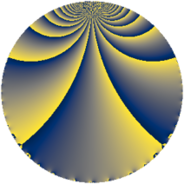# Properties

 Label 78.2.kLevel $78$ Weight $2$ Character orbit 78.k Rep. character $\chi_{78}(11,\cdot)$ Character field $\Q(\zeta_{12})$ Dimension $16$ Newform subspaces $1$ Sturm bound $28$ Trace bound $0$

# Related objects

## Defining parameters

 Level: $$N$$ $$=$$ $$78 = 2 \cdot 3 \cdot 13$$ Weight: $$k$$ $$=$$ $$2$$ Character orbit: $$[\chi]$$ $$=$$ 78.k (of order $$12$$ and degree $$4$$) Character conductor: $$\operatorname{cond}(\chi)$$ $$=$$ $$39$$ Character field: $$\Q(\zeta_{12})$$ Newform subspaces: $$1$$ Sturm bound: $$28$$ Trace bound: $$0$$

## Dimensions

The following table gives the dimensions of various subspaces of $$M_{2}(78, [\chi])$$.

Total New Old
Modular forms 72 16 56
Cusp forms 40 16 24
Eisenstein series 32 0 32

## Trace form

 $$16 q + 8 q^{7} + O(q^{10})$$ $$16 q + 8 q^{7} - 24 q^{10} - 24 q^{13} + 8 q^{16} - 16 q^{19} - 24 q^{21} - 8 q^{28} + 24 q^{30} + 16 q^{31} - 24 q^{33} + 24 q^{34} + 24 q^{36} + 16 q^{37} + 48 q^{39} + 24 q^{45} + 24 q^{46} + 24 q^{49} - 8 q^{52} - 24 q^{55} - 24 q^{57} - 24 q^{60} - 24 q^{61} - 24 q^{63} - 48 q^{66} + 32 q^{67} - 48 q^{69} - 24 q^{72} + 56 q^{73} - 16 q^{76} - 96 q^{79} + 24 q^{81} - 48 q^{82} - 24 q^{85} + 48 q^{87} - 16 q^{91} - 24 q^{93} - 24 q^{94} + 16 q^{97} + O(q^{100})$$

## Decomposition of $$S_{2}^{\mathrm{new}}(78, [\chi])$$ into newform subspaces

Label Dim $A$ Field CM Traces $q$-expansion
$a_{2}$ $a_{3}$ $a_{5}$ $a_{7}$
78.2.k.a $16$ $0.623$ 16.0.$$\cdots$$.9 None $$0$$ $$0$$ $$0$$ $$8$$ $$q+(-\beta _{7}-\beta _{15})q^{2}+(-\beta _{1}-\beta _{3}-\beta _{4}+\cdots)q^{3}+\cdots$$

## Decomposition of $$S_{2}^{\mathrm{old}}(78, [\chi])$$ into lower level spaces

$$S_{2}^{\mathrm{old}}(78, [\chi]) \cong$$ $$S_{2}^{\mathrm{new}}(39, [\chi])$$$$^{\oplus 2}$$